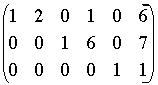SEARCH HOMEMath Central Quandaries & QueriesQuestion from Rasanga, a student: Use the row echelon form of the augmented matrix to solve the following linear system. X1+2X2+X4=6 X3+6X4=7 X5=1Rasanga,

The augmented matrix is already in row echelon formFrom this, or the equations you have, you can read off the solution

x5 = 1
x3 = 7 - 6x4
x1 = 6 - 2x2 - x4

There are in fact infinitely many solutions since x2 and x4 can be given any values and the x1, x3 and x5 generated by the expressions above.

HarleyMath Central is supported by the University of Regina and The Pacific Institute for the Mathematical Sciences.